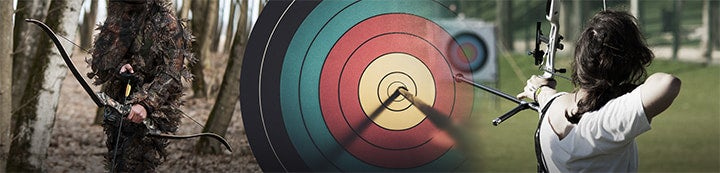1 - 4 of 4 Posts

·
##### Registered
Joined
·
89 Posts
Discussion Starter · ·
Any one out there have any archery calculations or formula for calculation anything to do with archery
(Arrow weight, FOC, Draw length, Bow energy, Arrow trajectory, etc).
I would like to compile a full collection of math formula to provide to members
So we could understand how and why it works.

Thanks#### beezaur

·
##### Registered
Joined
·
2,425 Posts
The following is a formula that gives arrow FOC in percent, as a function of component weights and some standard measurements.

FOC = 50*(2*df*wf-l*(wb+wf-wi+wn-wp)+dn*(wb+wf+wi+wp+l*mu))/((dn+l)*(wb+wf+wi+wn+wp+l*mu)),

where wp is the weight of the point, wi is the weight of the point insert, mu is the weight per unit length of the bare shaft, l is the length of the bare shaft, wf is the total weight of the fletching, df is the distance from the nock end of the bare shaft to the center of mass of the fletching, wb is the weight of the nock bushing (or pin), wn is the weight of the nock, and dn is the distance between the string position in the nock and the beginning of the shaft proper.

This formula was developed using the principles of statics, namely the summation of moments about some arbitrary point. The general procedure is fully explained in any engineering statics text.

The formula is good for field points and other points that have their center of mass near the end of the shaft. FOC for broadheads would be slightly different.

The formula is most conveniently implemented using a spreadsheet or programmable calculator. A pretty decent (and free) spreadsheet is available at: www.openoffice.org.

Scott

PS: you can use any units (grams, grains, inches, mm, whatever) so long as you don't mix them. For example, arrow weight in grains per inch will give a bad FOC if used with point weight in grams of arrow length in mm.

#### clayking

·
##### Occam's Razor
Joined
·
9,326 Posts
There is an article in the current issue of "Archery Focus" on "cant" and why it works etc. Not sure it's a pure math equation, but it played a role.

I am sure that the makers of the archery software programs could fill your boots with plenty of them..........ck

#### PSI

·
##### Registered
Joined
·
123 Posts
The book...

The Physical Laws of Archery, by T.L. Liston

...has what you're looking for. Many of the formulas are for arrow flight without friction or drag, but can be modified to incorporate that if you so desire. There is also a pretty god explanation on force draw curves and how bows store energy.

1 - 4 of 4 Posts